Courses

# Short Answer Type Questions(Part- 1)- Coordinate Geometry Class 10 Notes | EduRev

## Class 10 : Short Answer Type Questions(Part- 1)- Coordinate Geometry Class 10 Notes | EduRev

The document Short Answer Type Questions(Part- 1)- Coordinate Geometry Class 10 Notes | EduRev is a part of the Class 10 Course Class 10 Mathematics by VP Classes.
All you need of Class 10 at this link: Class 10

Q1. Points P (5, − 3) is one of the two points of trisection of the line segment joining the points A (7, − 2) and B (1, − 5) near to A. Find the coordinates of the other point of trisection.

Sol.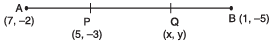∴ other point Q is the mid point of PB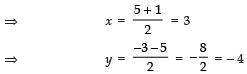Thus, the point Q is (3, − 4)

Q2. Find the area of the quadrilateral ABCD whose vertices are A (1, 0), B (5, 3), C (2, 7) and D (− 2, 4).

Sol. Area of ΔABC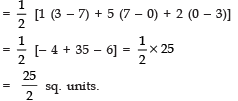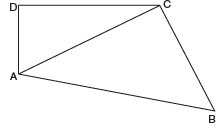Area of Δ ACD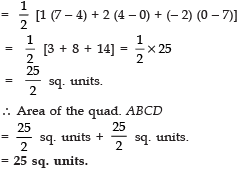Q3. Points P, Q, R and S, in this order, divide a line segment joining A (2, 6), B (7, − 4) in five equal parts. Find the coordinates of P and R.

Sol.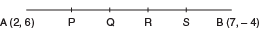∵ P, Q, R and S divide AB in five equal parts.
∴ AP = PQ = QR = RS = SB
Now, P divides AB in the ratio 1 : 4
∴ Coordinates of P are: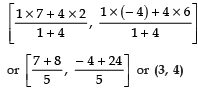Again, R divides AB in the ratio 3 : 2
∴ Coordinates of R are: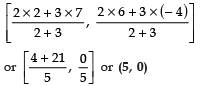Q4. A (− 4, − 2), B (− 3, − 5), C (3, − 2) and D (2, k) are the vertices of a quad. ABCD. Find the value of k, if the area of the quad is 28 sq. units.

Sol. Area of quad ABCD = 28 sq. units
∴ [ar (Δ ABD)] + [ar (Δ BCD)] = 28 sq. units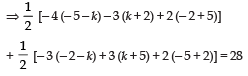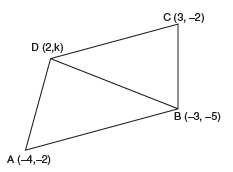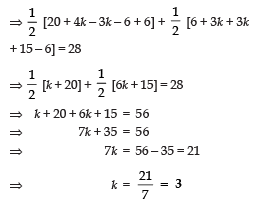Q5. Find the point on y-axis which is equidistant from the points (5, − 2) and (− 3, 2).

Sol. Let the required point be P (0, y) ä The given points are A (5, − 2) and B (− 3, 2)
∴ PA = PB
⇒ PA2 = PB2
∴(5 − 0)2 + (− 2 − y)2 =(− 3 − 0)2 + (2 − y)2
⇒ 52 + (− 2 − y)2 = (− 3)2 + (2 − y)2
⇒ 25 + 4 + y2 + 4y = 9 + 4 + y2 − 4y
⇒ 25 + 4y = 9 − 4y
⇒ 8 y = − 16 ⇒ y = − 2

Thus, the required point is (0, − 2)

Q6. Find the point on y-axis which is equidistant from (− 5, 2) and (9, − 2).

Sol. Let the required point on Y-axis be P (0, y).

The given points are A (− 5, 2) and B (9, − 2)

∴ AP = BP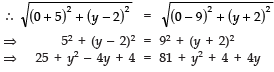⇒ − 4y − 4y = 81 + 4 − 4 − 25
⇒ − 8y =56

⇒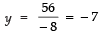∴ The required point = (0, −7)

Q7. Find the value of x for which the distance between the points P (4, − 5) and Q (12, x) is 10 units.

Sol. The given points are P (4 − 5) and Q (12, x) such that PQ = 10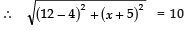⇒ (12 − 4)2 + (x + 5)2 =102
⇒ 82 + (x + 5)2 = 100
⇒ 64 + x2 + 25 + 10x = 100
⇒ x2 + 10x − 11 = 0
⇒ (x − 1) (x + 11) = 0
⇒ x = 1 or x = −11

Q8. Find the relation between x and y if the points (2, 1), (x, y) and (7, 5) are collinear.

Sol. Here, x1 = 2, y1 = 1
x2 = x, y2 = y
x3 = 7, y3 = 5

∴ Area of triangle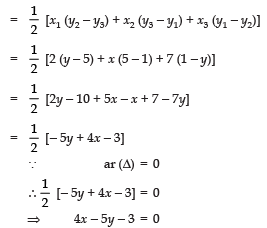which is the required relation.

Q9. If A (− 2, 4), B (0, 0) and C (4, 2) are the vertices of Δ ABC, then find the length of the median through the vertex A.

Sol. ∵ AD is the median on BC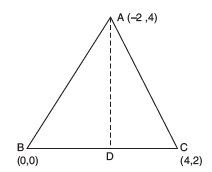∴ D is the mid-point of BC.
⇒ Coordinates of D are: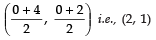Now, the length of the median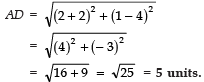Q10. If the points A (4, 3) and B (x, 5) are on the circle with the centre O (2, 3), find the value of x.

Sol. Let O (2, 3) be the centre of the circle.
∴ OA = OB ⇒ OA2 = OB2
⇒ (4 − 2)2 + (3 − 3)2 =(x − 2)2 + (5 − 3)2
⇒ 22 =(x − 2)2 + 22
⇒ (x − 2)2 = 0
⇒ x −2 = 0
⇒ x = 2

Thus, the required value of x is 2.

Q11. If the vertices of a Δ are (2, 4), (5, k) and (3, 10) and its area is 15 sq. units, then find the value  of ‘k’.

Sol. The area of the given Δ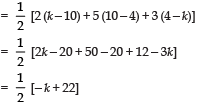But ar (Δ) = 1 5    [given]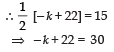⇒ − k = 30 − 22 = 8
⇒ k = −  8

Q12. The vertices of a triangle are: (1, k), (4, − 3), (− 9, 7) and its area is 15 sq. units. Find the value of k.

Sol. Area of the given triangle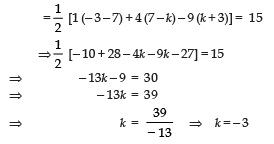Q13. Find the area of a ΔABC whose vertices are A (− 5, 7), B (− 4, − 5) and C (4, 5).

Sol. Here, x1 = − 5, y1 = 7
x2 = − 4, y2 = − 5
x3 =4,  y3 = 5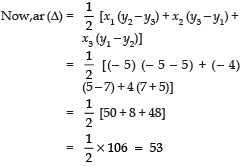∴ The required ar (Δ ABC) = 53 sq. units.

Q14. Find the value of k such that the points (1, 1), (3, k) and (− 1, 4) are collinear.

Sol. For the three points, to be collinear, the area of triangle formed by them must be zero.
∴ Area of triangle = 0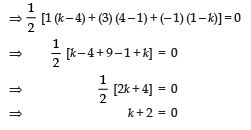⇒ k = - 2

Q15. For what value of p, the points (− 5, 1), (1, p) and (4, − 2) are collinear?

Sol. Since the points are collinear,
∴ The area of the Δ formed by these points must be zero.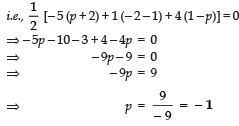Q16. For what value of p, are the points (2, 1), (p, − 1) and (− 1, 3) collinear?

Sol. ∵ The given points are collinear.
∴ The area of a triangle formed by these points must be zero. i.e.,Area of triangle = 0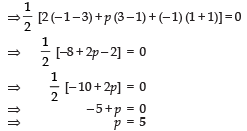Q17. Find the ratio in which the line 3x + 4y − 9 = 0 divides the line segment joining the points (1, 3) and (2, 7).

Sol. Let the ratio be k : 1.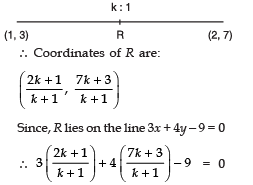⇒ 6k + 3 + 28k + 12 − 9k + 9 = 0
⇒ (6k + 28k − 9k) + (3 + 12 − 9) = 0
⇒ 25k + 6 = 0

⇒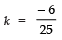∴ The required ratio is − 6 : 25 or 6 : 25

Q18. If the point P (x, y) is equidistant from the points A (3, 6) and B (− 3, 4), prove that 3x + y − 5 = 0.

Sol.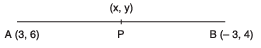∵ P is equidistant from A and B.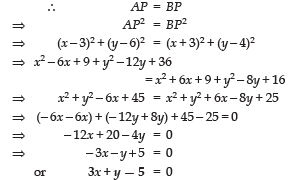Q19. The coordinates of A and B are (1, 2) and (2, 3). If P lies on AB, then find the coordinates of P such that: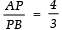Sol.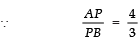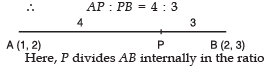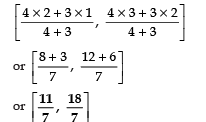Q20. If A (4, − 8), B (3, 6) and C (5, − 4) are the vertices of a Δ ABC, D(4, 1) is the mid-point of BC and P is a point on AD joined such that  AP/PD = 2 , find the coordinates of P.

Sol. ∵ D is the mid-point of B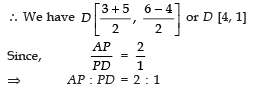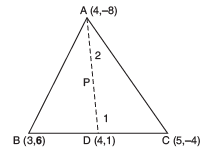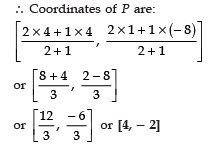Offer running on EduRev: Apply code STAYHOME200 to get INR 200 off on our premium plan EduRev Infinity!

132 docs

,

,

,

,

,

,

,

,

,

,

,

,

,

,

,

,

,

,

,

,

,

;